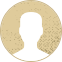Top Special Offer! Check discount

Get 13% off your first order - useTopStart13discount code now!

# Median, Mode, and Mean

133 views 2 pages ~ 400 words
Get a Custom Essay Writer Just For You!

Experts in this subject field are ready to write an original essay following your instructions to the dot!

The mean, commonly known as the average, is calculated by dividing the total number of values in a given data collection by the number of values (n). The median is the midpoint of a set of data that has been ordered either from smallest to largest or from largest to smallest. In contrast, mode refers to the value that appears the most frequently in a given data set. Under different circumstances, the mean, median, and mode are all reliable indicators of central tendency. For particular demographics, some become more appropriate than others. This essay explores the traits of a population for which the usage of mean, median, and mode would be most and least appropriate. The mean can be applied where the population data is either discrete or continuous data set (Laerd, 2017). However, most of the time the mean is used with continuous data. It gives the most common value in a data set. It is appropriate for populations that have no outliners since it reduces any errors in the prediction if the data set. It is the most commonly known measure of central tendency. However, is prone to the effect of the outliners. Outliners are the unusual values compared to the rest of the values in the data set (Laerd, 2017). Therefore, it is not appropriate for populations that have outliners. In such cases, the median is the best to use. In addition to that, populations with skewed data set make the use of mean inappropriate.

The median works well when the number of values in the data set is an odd number (Laerd, 2017). For example, seven, nine, fifteen etc. It is appropriate where the given data set in population is skewed. However, it is not appropriate where the number of values in a data set are even, such as six, eight, sixteen etc. On the other hand, the mode works well where the population data is categorical and where we want to determine the most common category (Laerd, 2017). It is appropriate where the data set for a given population is skewed. It is inappropriate for population data set where the most common mark lies far much away from the other values in the population data set. In such cases, it does not give a very good measure of central tendency in cases where.

References

April 06, 2023
Category:
Subject area:
Number of pages

2

Number of words

400

36Rate:

4.7

Expertise Value
Verified writer

Richard is the best writer for Data Science tasks, even if you have something really complex. I needed to do tasks on security matters and already had a draft. Sharing my ideas with Richard ended up in a perfect paper!

Hire Writer

This sample could have been used by your fellow student... Get your own unique essay on any topic and submit it by the deadline.

Eliminate the stress of Research and Writing!

Hire one of our experts to create a completely original paper even in 3 hours!